PTC2.5K-CH
PTC5K-CH
PTC10K-CH
PTC10K-SL

## Question

How do I modify the default remote SET T voltage in the PTCxx-CH?

The PTCxx-CH includes a safety circuit to protect the laser or thermoelectric in case the external setpoint signal is lost. If the EXT SET T drops below the trigger voltage, a default safe setpoint voltage is activated.

Figure 1. EXT SET T Example Circuit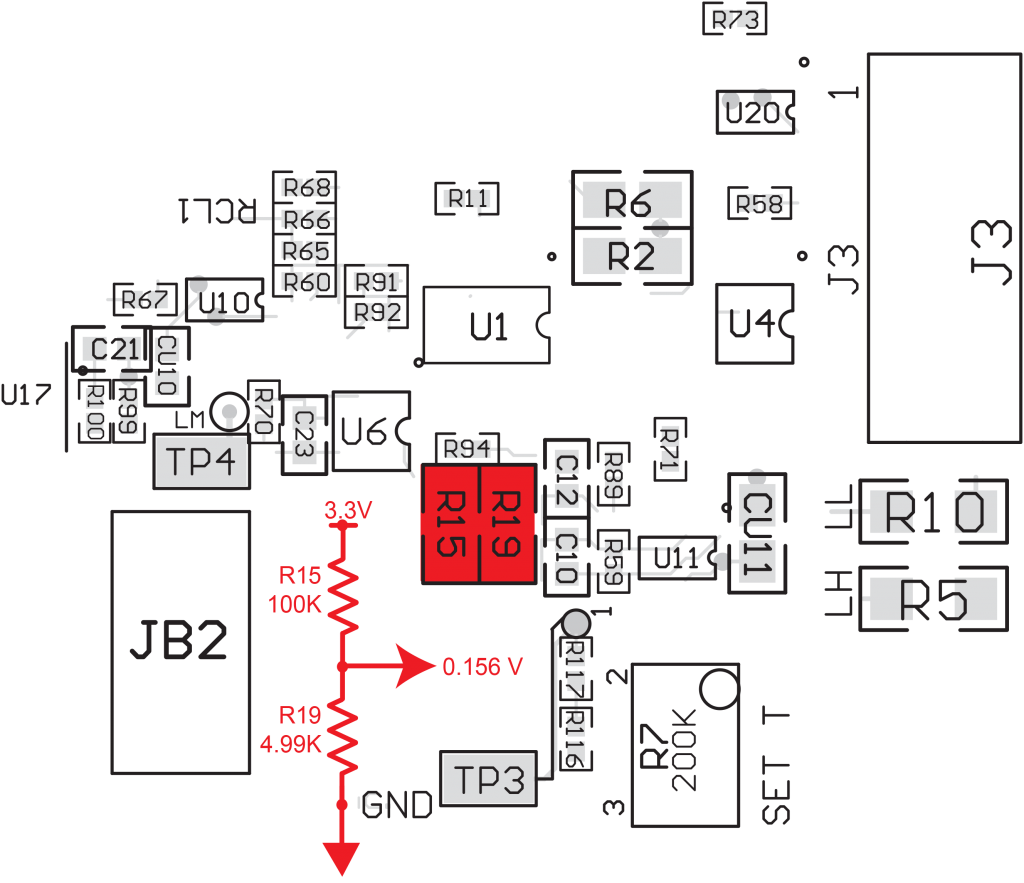The divider string circuit for setting up the EXT SET T trigger voltage is shown in Figure 1. When the default voltage is 0.156 V, it then is multiplied by 2 down circuit, setting up the actual default trigger voltage of 0.312 V.

The resistors are 1206 SMTs and can be changed in the field.

The equations are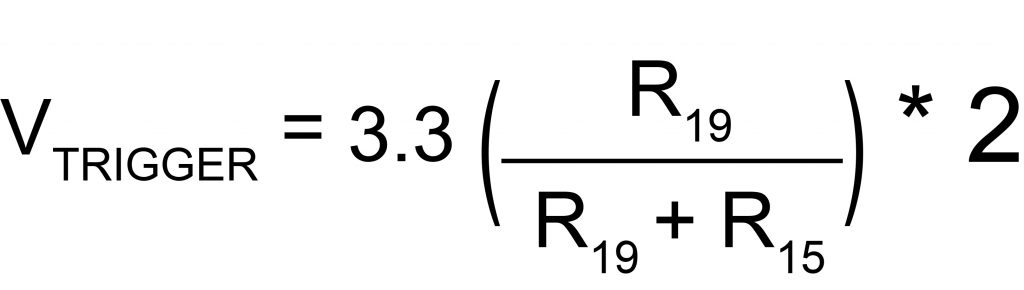and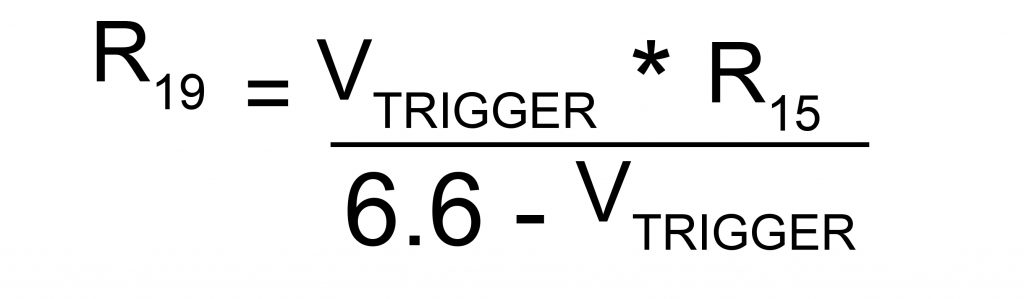NOTE: It is preferable to leave R15 at 100K and only change R19.

Figure 2. Safety Default Example Circuit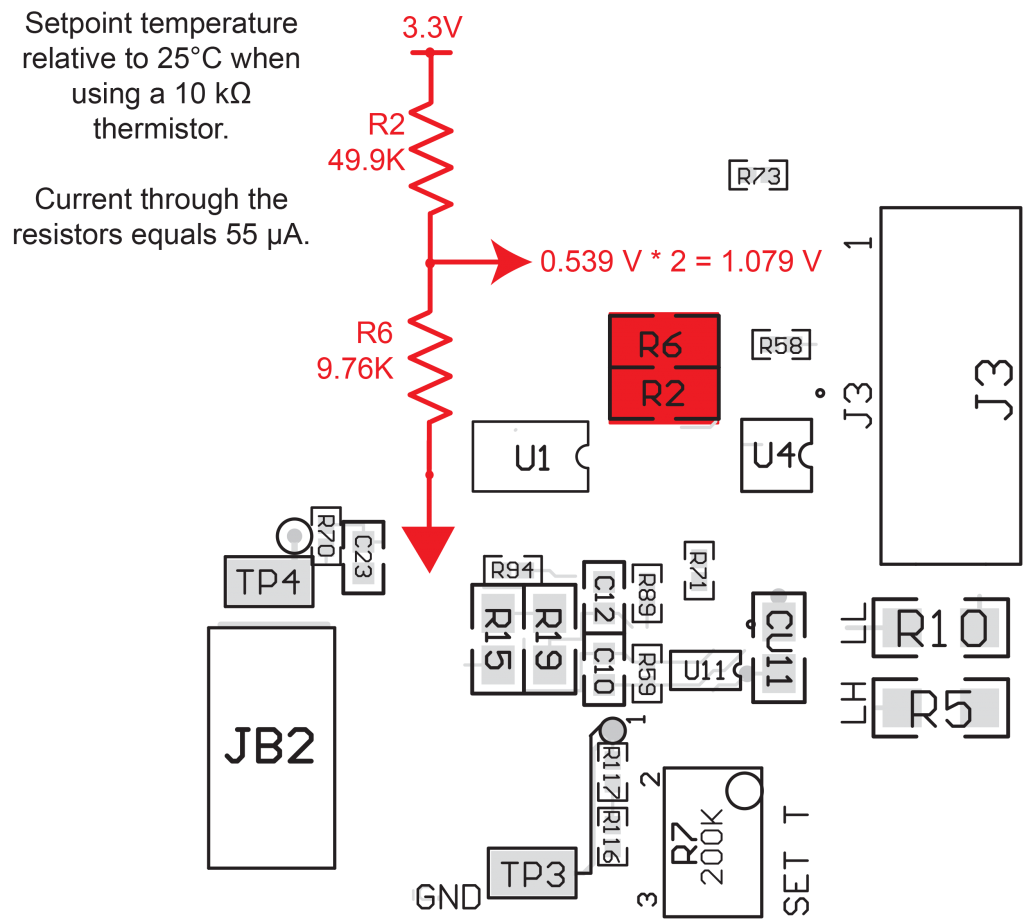The divider string circuit for the safety default setpoint temperature voltage (Figure 2) activates when the EXT SET T voltage drops below 0.312 V and the system substitutes the voltage across R6 as the setpoint. The default voltage is 0.539 V and is multiplied by 2 down circuit, providing a setpoint voltage of 1.079 V. When using a 10 kΩ thermistor and 100 µA bias current, this is about 25°C.

The resistors are 1206 SMTs and can be changed in the field. Current through the resistors is about 55 µA.

The equations are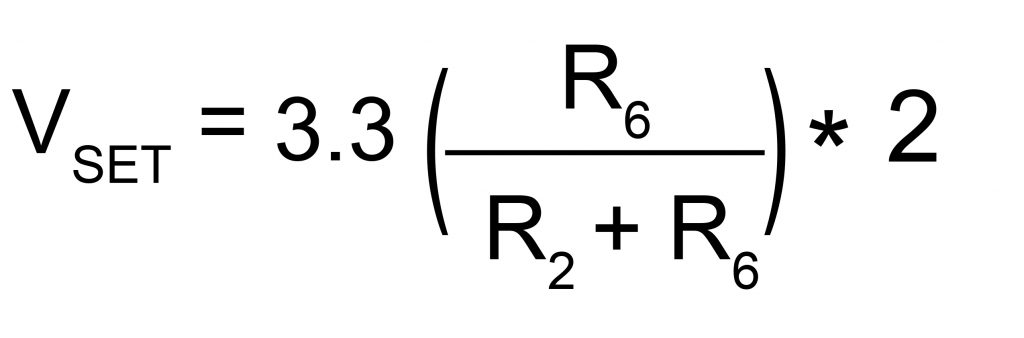andNOTE: It is preferable to leave R2 at 49.9K and only change R6.